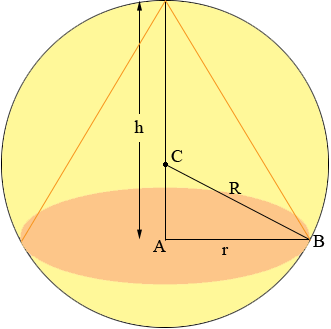D

#### Largest volume of an inscribed cone in a sphere

6 viewed last edited 3 years agoAnonymous
0We solved the volume of largest cylinder inscribed in a circle in class. We wrote a function and looked for the peak by graphing the function on the calculator. I was wondering if we can take the same approach for finding the largest volume of a cone inscribed in a cylinder. How would we go about doing it?Mahesh Godavarti
1
Let's split this into two problems. 1. Where h < R . Then using Pythagoras' theorem (R - h)^2 + r^2 = R^2 . 2. Where h \geq R . The using Pythagoras' theorem (h-R)^2 + r^2 = R^2 . In both cases, the relationship is essentially (h - R)^2 + r^2 = R^2 . So, we can treat both problems as a single problem. The volume of a cone is \frac{1}{3} \pi r^2 h = \frac{1}{3} \pi \left( R^2 - (h-R)^2 \right) h = \frac{1}{3} \pi (h^3 - 2h^2 R). Note that 0 \leq h \leq 2R . Graph the function V(h) = \frac{1}{3} \pi (h^3 - 2h^2 R) using your TI-84 CE PLUS calculator and find the maximum in the domain of interest.